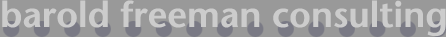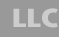### Determining sample size: an example

Suppose you are investigating mean personal income. You want to determine the sample size needed so that your sample estimate is within \$500 of the true population value. Furthermore, you want to be 95% confident that the sample mean falls within \$500 of the actual, unknown population mean. Now you need an estimate of the variance in the population. Some a priori knowledge of the subject matter is often sufficient to guide this judgment. Based on past studies you have read and your own experience, you make a judgment about the size of the population standard deviation: \$1,000.

Confidence level: 95%

Desired precision: +/- \$500

Estimated standard deviation: +/-\$1,000

Required sample size: 15.37 or 16

Wow! You only need a sample size of 16 to estimate mean personal income when the population standard deviation is \$1000 and allowed precision is \$500. But suppose you want to be more precise, say twice as precise? \$500 is not a very precise interval to use, relative to your estimated standard deviation of \$1,000. You accomplish this by halving the width of the desired precision interval. Then,

Confidence level: 95%

Desired precision: +/- \$250

Estimated standard deviation: +/-\$1,000

Required sample size: 61.47 or 62

Notice the trade-off; doubling your precision increased your sample size by nearly a factor of four. Whenever precision is increased by a factor of x, sample size is increased by a factor of x2.

Some practical hints include assuming the worst-case scenario in order to be conservative with your estimates of sample size. One way to accomplish this--the example is particularly simple using proportional data--is to assume maximum variability or disagreement (i.e. a 50/50 split).

Back to sample size tools

email bfc | phone 608.220.1639